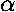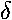## Horizon and Equator Coordinate Systems

### Explanation of Terms

#### CELESTIAL SPHERE

sphere:
a surface in three-dimensional space all of whose points are equidistant from a fixed point (the center).
great circle:
a circle on the surface of a sphere which divides the sphere into two equal parts.
small circle:
any circle on the surface of a sphere which is not a great circle.
celestial sphere:
a model of the sky as a spherical shell. All celestial bodies (Sun, Moon, stars,...) are considered located on the celestial sphere. It has the following properties:
1. It is imaginary.
2. It has an indefinite radius large enough that its center may be anywhere at all. Its center is often taken as the center of the Earth. Since the offset between the center of the Earth and the Earth's surface is ``small,'' the celestial sphere's center could also be taken at an observer's position.
3. Although objects are various distances from Earth, each is projected onto the sphere so that each star has an apparent place on the sphere. Relative posistions on the sphere are specified by angles.

#### HORIZON SYSTEM

zenith:
the direction straight up, i.e., directly overhead.
the direction diametrically opposite to the zenith.
horizon:
1. the great circle midway between zenith and nadir 2. the great circle formed by the intersection of the celestial sphere with a plane perpendicular to the line from an observer to the zenith.
meridian:
the great circle passing through the observer's zenith, and north and south points on the horizon. It is both a vertical circle and an hour circle. The observer's meridian is the most important of all circles of reference.
vertical circle:
any great circle passing through both the observer's zenith and nadir. Vertical circles receive their name from the fact that they are perpendicular to the horizon.
altitude:
the angle from the horizon along the vertical circle to the object.
azimuth:
the angle from the north point of the horizon clockwise to the foot of the vertical circle through the object.
transit:
when a star crosses the observer's meridian; a.k.a.culminate

#### EQUATOR SYSTEM

celestial pole:
one of the two points determined by extending the Earth's axis of rotation to the celestial sphere. The north and south celestial poles are the centers of rotation of the celestial sphere.
celestial equator:
1. the great circle on the celestial sphere midway between the north and south celestial poles. 2. the great circle on the celestial sphere determined by extending the Earth's equator to the celestial sphere.
diurnal circle:
the apparent path of a star in the sky during one day due to the the rotation of the Earth. Diurnal circles are parallel to the celestial equator.
hour circle:
any great circle on the celestial sphere which passes through the north and south celestial poles.
vernal equinox:
the point where the Sun crosses the celestial equator from south to north each year. Also known as the First Point of Aries.
right ascension:
the angle measured in units of time $15° = 1h$ eastward along the celestial equator from the vernal equinox to the foot of the hour circle through the object. Symbol:or $\alpha$ or R.A.
declination:
the angle between a object on the celestial sphere and the celestial equator, measured north or south along the hour circle through the object. Symbol:or $\delta$ or dec.
hour angle:
the angle westward along the celestial equator from the observer's meridian to the hour circle through the object. Symbol: t or H.A.
ecliptic:
the apparent path of the Sun on the celestial sphere over a year.